Foxtable(狐表)用户栏目专家坐堂 → 用控件控制调用

共有800人关注过本帖树形打印复制链接

# 主题：用控件控制调用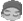yangwenghd
1楼 | 信息 | 搜索 | 邮箱 | 主页 | UC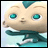加好友发短信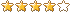If e.Form.Controls("ComboBox1").Text = "方式一" Then    If e.DataCol.Name = "产品编号" Then        Dim dr As DataRow        dr = DataTables("库存").Find("属性_产品编号= '"  & e.DataRow("产品编号") & "'" )        If dr IsNot Nothing '如果找到, 则设置各列内容            e.DataRow("产品信息_大类别")= dr("属性_大类别")        End If    End If  '得到产品信息End If能帮忙看看这个吗 感谢 感谢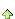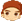y2287958
2楼 | 信息 | 搜索 | 邮箱 | 主页 | UC加好友发短信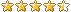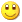Post By：2018/11/9 11:10:00 [只看该作者]

 If e.Form.Controls("ComboBox1").Text = "方式一" Then    Dim r As Row = CurrentTable.Current    If r IsNot Nothing        Dim dr As DataRow        dr = DataTables("库存").Find("属性_产品编号= '"  & r("产品编号") & "'" )        If dr IsNot Nothing '如果找到, 则设置各列内容            r("产品信息_大类别")= dr("属性_大类别")        End If    End If  '得到产品信息End If3楼 | 信息 | 搜索 | 邮箱 | 主页 | UC加好友发短信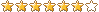Post By：2018/11/9 11:21:00 [只看该作者]

 看2楼关键代码       Dim r As Row = Tables("表A").Current     If r IsNot Nothingyangwenghd
4楼 | 信息 | 搜索 | 邮箱 | 主页 | UC加好友发短信Post By：2018/11/9 11:49:00 [只看该作者]

 感谢 感谢  能再请教一下老师吗？感谢 If e.Form.Controls("ComboBox1").Text = "方式一" Then    Dim r As Row = CurrentTable.Current    If r IsNot Nothing        Dim dr As DataRow        dr = DataTables("运费录入库").Find("国家英= '"  & r("国家信息_国家英语") & "'" )        If dr IsNot Nothing '如果找到, 则设置各列内容            r("方式一_物流数据_价格")= dr("价格")            r("方式一_物流数据_挂号费")= dr("挂号费")        End If    End IfEnd If  '得到产品信息  可以是点击后一整列都执行吗？不是只有选中的行，感谢 感谢5楼 | 信息 | 搜索 | 邮箱 | 主页 | UC加好友发短信Post By：2018/11/9 11:58:00 [只看该作者]

 If e.Form.Controls("ComboBox1").Text = "方式一" Then    For Each r As Row In Tables("表A").Rows        Dim dr As DataRow        dr = DataTables("运费录入库").Find("国家英= '"  & r("国家信息_国家英语") & "'" )        If dr IsNot Nothing '如果找到, 则设置各列内容            r("方式一_物流数据_价格")= dr("价格")            r("方式一_物流数据_挂号费")= dr("挂号费")        End If    NextEnd Ifyangwenghd
6楼 | 信息 | 搜索 | 邮箱 | 主页 | UC加好友发短信Post By：2018/11/9 12:04:00 [只看该作者]

 看懂了，老师 ，谢谢 [此贴子已经被作者于2018/11/9 12:05:06编辑过]